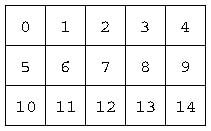BFOIT - Introduction to Computer Programming

# Why Count Starting With Zero?

1. In GridToolkit, rows and columns were numbered starting with zero instead of one.  The index that is used to identify a particular cell also started with the first cell numbered zero. This convention has been in place forever in the world of computer programming.

The reason is that this simplifies the code.  Can you find the code in our GridWorld which is simpler than it would have to be if we started numbering the index at one instead of zero?  How would the code need to be changed if the base was one instead of zero?

The Bottom Line - by having cell numbers, column numbers, and row numbers start at zero, computing the location of cells is simpler.`````` ; move the turtle to top-left corner of specified cell to gridGotoCell :idx gridGotoTopLeft setheading 180 forward product gridCellSize (int quotient :idx gridNumCol)    setheading 90 forward product gridCellSize (remainder :idx gridNumCol) end ``````

Given the grid above and the gridTotoCell procedure which moves the turtle to a specified cell, let's look at the code and work through a couple examples.  First let's look at the edge cases.

1. First example: idx = 0, gridNumCol = 5,
Both forward instructions will result in no movement which is exactly what we want.  Zero divided by anything results in zero for a quotient and also results in a zero remainder.  So, the product of gridCellSize and zero always produces zero.
2. Second example: idx = 14, gridNumCol = 5,
The first forward, moving south, starts with quotient 14 5 outputing 2.8.  int with 2.8 as its input, outputs 2.  This is exactly what we want, the turtle moves south two cells.
The second forward, now moving east, has remainder 14 5 outputing 4.  This works too, moving the turtle to the east four cells.

So, how would this code need to change if we started our indexing at one instead of zero?  Answer: we would have to subtract one from the index before using it. Here is what the forward instructions would look like.

```  forward product gridCellSize (int quotient (difference :idx 1) gridNumCol)
forward product gridCellSize (remainder (difference :idx 1) gridNumCol)
```

Once again, the bottom line is that the code is simpler when cell numbers, column numbers, and row numbers start out at zero instead of one.